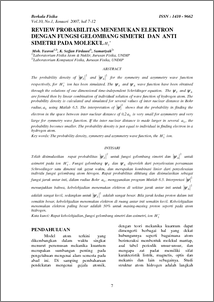# REVIEW PROBABILITAS MENEMUKAN ELEKTRON DENGAN FUNGSI GELOMBANG SIMETRI DAN ANTI SIMETRI PADA MOLEKUL H2+

Ketut, Sofjan Firdausi and Sumariyah, Sumariyah and Yusron, Muhamad (2007) REVIEW PROBABILITAS MENEMUKAN ELEKTRON DENGAN FUNGSI GELOMBANG SIMETRI DAN ANTI SIMETRI PADA MOLEKUL H2+. BERKALA FISIKA, 10 (1). pp. 7-12. ISSN 1410 - 9662Preview
PDF - Published Version
238Kb

## Abstract

The probability density of 2 S y and 2 A y for the symmetry and asymmetry wave function respectively, for + 2 H ion has been simulated. The S y and A y wave function have been obtained through the solutions of one dimensional time-independent Schrödinger equation. The S y and A y are formed then by linear combination of individual solution of wave function of hydrogen atom. The probability density is calculated and simulated for several values of inter nuclear distance in Bohr radius, 0 a using Matlab 6.5. The interpretation of 2 y shows that the probability in finding the electron in the space between inter nuclear distance of 0,2 0 a is very small for asymmetry and very large for symmetry wave function. If the inter nuclear distance is made larger in several 0 a , the probability becomes smaller. The probability density is just equal to individual in finding electron in a hydrogen atom.

Item Type: Article The probability density, symmetry and asymmetry wave function, the H2+ ion. Q Science > QC Physics Faculty of Science and Mathematics > Department of Physics 2038 INVALID USER 02 Dec 2009 10:34 02 Dec 2009 10:34

Repository Staff Only: item control page